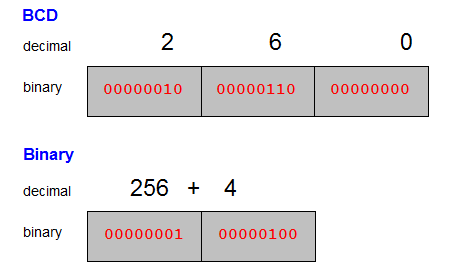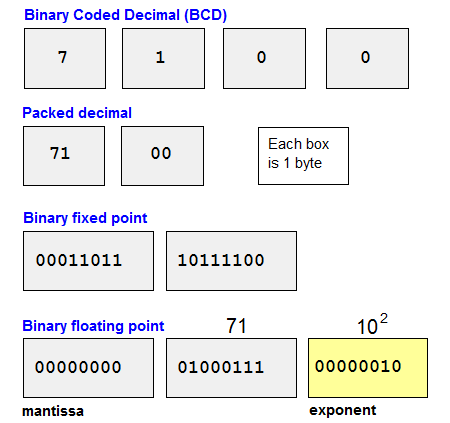binary coded decimal

(redirected from binary coded decimals)
Also found in: Dictionary.

binary coded decimal

(data)
(BCD, packed decimal) A number representation where a number is expressed as a sequence of decimal digits and then each decimal digit is encoded as a four-bit binary number (a nibble). E.g. decimal 92 would be encoded as the eight-bit sequence 1001 0010.

In some cases, the right-most nibble contains the sign (positive or negative).

It is easier to convert decimal numbers to and from BCD than binary and, though BCD is often converted to binary for arithmetic processing, it is possible to build hardware that operates directly on BCD.

BCD

(Binary Coded Decimal) The storage of numbers in which each decimal digit is converted into a binary number and stored in a single 8-bit byte. For example, a 12-digit decimal number would be represented as 12 bytes. BCD uses more storage for numbers than binary encoding (see below). Prior to 8-bit bytes in the 1960s, BCD encoding used 6-bit characters. See binary numbers and byte.

BCD and BinaryThe BCD method codes each decimal digit in binary and stores it in its own byte. The binary method converts the entire decimal number into a binary number. In the binary example above, the 1 in the left byte is the ninth bit starting from the rightmost bit in the right byte (1-2-4-8-16-32-64-128-256-512-1024-2048-4096-8192-16384-32768).

How Numbers Are StoredBCD is one of four primary ways numbers are stored in the computer.
Copyright © 1981-2019 by The Computer Language Company Inc. All Rights reserved. THIS DEFINITION IS FOR PERSONAL USE ONLY. All other reproduction is strictly prohibited without permission from the publisher.
Site: Follow: Share:
Open / Close